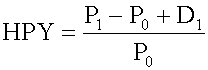Tags
Question
Holding period yield (HPY) formula:

HPY= [...]Tags
Question
Holding period yield (HPY) formula:

HPY= [...]

?

Tags
Question
Holding period yield (HPY) formula:

HPY= [...]If you want to change selection, open document below and click on "Move attachment"

Subject 4. Different Yield Measures of a U.S. Treasury Bill
tead, return on investment should be measured based on cost of investment. It is annualized using a 360-day year, not a 365-day year. It annualizes with simple interest and ignores the effect of interest on interest (compound interest). <span>Holding period yield (HPY) is the return earned by an investor if the money market instrument is held until maturity: P 0 = the initial price of the instrument P 1 = the price received for the instrument at its maturity D 1 = the cash distributio

#### Summary

status measured difficulty not learned 37% [default] 0

No repetitions